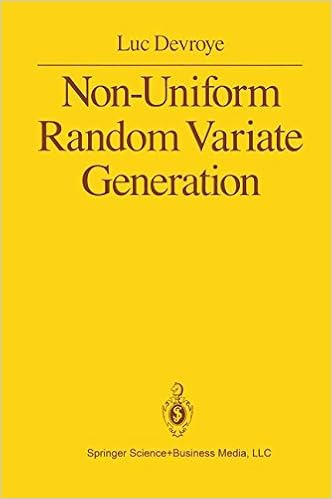## wrong algebra for slice sampler

Posted in Books, Kids, R, Statistics with tags , , , , , , , , , , , , on January 27, 2021 by xi'anOnce more, and thrice alas!, I became aware of a typo in our “Use R!” book through a question on X validated from a reader unable to reproduce the slice of a basic 2D slice sampler for a logistic regression with coefficients (a,b). Indeed, our slice reads as the incorrect set (missing the i=1,…,n)$\left\{ (a,b): y_i(a+bx_i) > \log \frac{u_i}{1-u_i} \right\}$

when it should have been$\bigcap_{i=1} \left\{ (a,b)\,:\ (-1)^{y_i}(a+bx_i) > \log\frac{u_i}{1-u_i} \right\}$

which is the version I found in my LaTeX file. So I do not know what happened (unless I corrected the LaTeX file at a later date and cannot remember it, but the latest chance on the file reads October 2011…). Fortunately, the resulting slices in a and b and the following R code remain correct. Unfortunately, both French and Japanese translations reproduce the mistake…## ratio-of-uniforms [#2]

Posted in Books, R, Statistics with tags , , , , , , on October 31, 2016 by xi'anFollowing my earlier post on Kinderman’s and Monahan’s (1977) ratio-of-uniform method, I must confess I remain quite puzzled by the approach. Or rather by its consequences. When looking at the set A of (u,v)’s in R⁺×X such that 0≤u²≤ƒ(v/u), as discussed in the previous post, it can be represented by its parameterised boundary

u(x)=√ƒ(x),v(x)=x√ƒ(x)    x in X

Similarly, since the simulation from ƒ(v/u) can also be derived [check Luc Devroye’s Non-uniform random variate generation in the exercise section 7.3] from a uniform on the set B of (u,v)’s in R⁺×X such that 0≤u≤ƒ(v+u), on the set C of (u,v)’s in R⁺×X such that 0≤u³≤ƒ(v/√u)², or on the set D of (u,v)’s in R⁺×X such that 0≤u²≤ƒ(v/u), which is actually exactly the same as A [and presumably many other versions!, for which I would like to guess the generic rule of construction], there are many sets on which one can consider running simulations. And one to pick for optimality?! Here are the three sets for a mixture of two normal densities:For instance, assuming slice sampling is feasible on every one of those three sets, which one is the most efficient? While I have no clear answer to this question, I found on Sunday night that a generic family of transforms is indexed by a differentiable  monotone function h over the positive half-line, with the uniform distribution being taken over the set

H={(u,v);0≤u≤h(f(v/g(u))}

when the primitive G of g is the inverse of h, i.e., G(h(x))=x. [Here are the slides I gave at the Warwick reading group on Devroye’s book last week:]

## precision in MCMC

Posted in Books, R, Statistics, University life with tags , , , , , , , , , on January 14, 2016 by xi'an

While browsing Images des Mathématiques, I came across this article [in French] that studies the impact of round-off errors on number representations in a dynamical system and checked how much this was the case for MCMC algorithms like the slice sampler (recycling some R code from Monte Carlo Statistical Methods). By simply adding a few signif(…,dig=n) in the original R code. And letting the precision n vary.

“…si on simule des trajectoires pendant des intervalles de temps très longs, trop longs par rapport à la précision numérique choisie, alors bien souvent, les résultats des simulations seront complètement différents de ce qui se passe en réalité…” Pierre-Antoine Guihéneuf

Rather unsurprisingly (!), using a small enough precision (like two digits on the first row) has a visible impact on the simulation of a truncated normal. Moving to three digits seems to be sufficient in this example… One thing this tiny experiment reminds me of is the lumpability property of Kemeny and Snell.  A restriction on Markov chains for aggregated (or discretised) versions to be ergodic or even Markov. Also, in 2000, Laird Breyer, Gareth Roberts and Jeff Rosenthal wrote a Statistics and Probability Letters paper on the impact of round-off errors on geometric ergodicity. However, I presume [maybe foolishly!] that the result stated in the original paper, namely that there exists an infinite number of precision digits for which the dynamical system degenerates into a small region of the space does not hold for MCMC. Maybe foolishly so because the above statement means that running a dynamical system for “too” long given the chosen precision kills the intended stationary properties of the system. Which I interpret as getting non-ergodic behaviour when exceeding the period of the uniform generator. More or less.